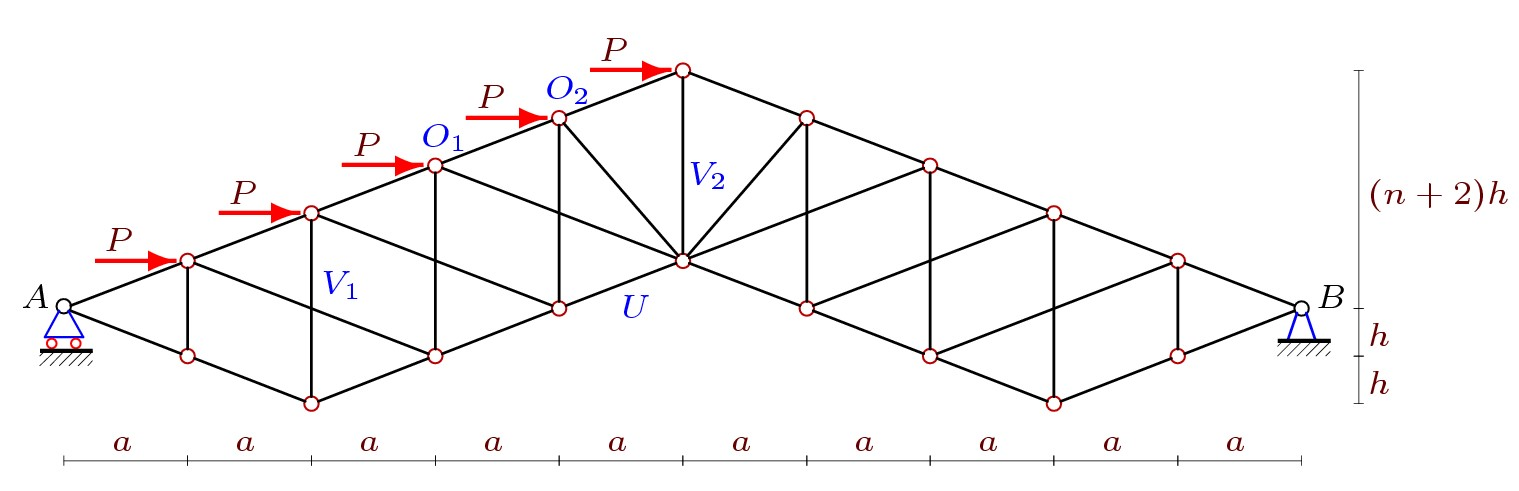# The formula for calculating the deformations of the arched truss with arbitrary number of panelsAuthors:
Abstract:

The scheme of statically determinate planar symmetric arched truss is proposed. Lattice of truss comprises a rack and braces. The derivation of the formula for the deflection of a truss under the influence of a load uniformly distributed over the nodes of the upper or lower belt, depending on the size of the truss and the number of panels is given. To summarize the number of solutions for trusses with a finite number of panels to the General case used the method of induction. All the transformations and solution of equilibrium equations of nodes are performed in the system of computer mathematics Maple. For finding recurrence equations satisfied by the coefficients in the formulas involves specialized operators of the system Maple. Asymptotic property of the solution is found out. Obtained and analyzed formula for forces in the most compression and tie rods.